Publicité

# So sánh cấu trúc protein_Protein structure comparison

20 May 2019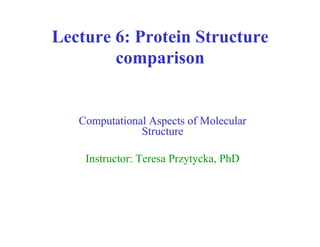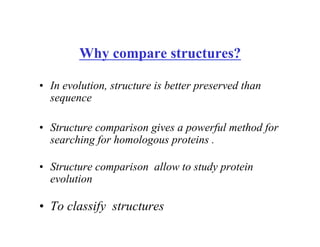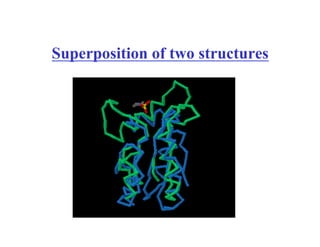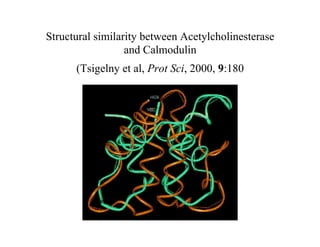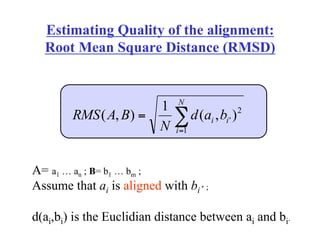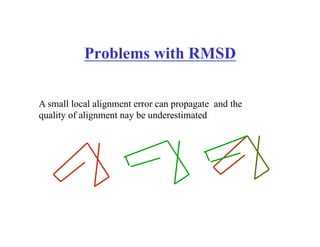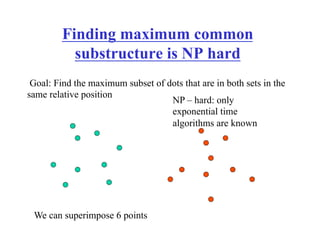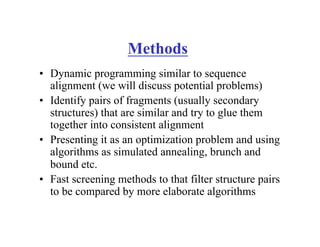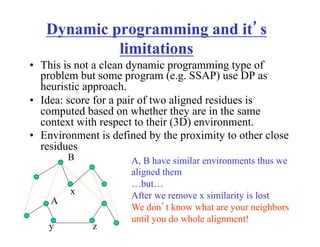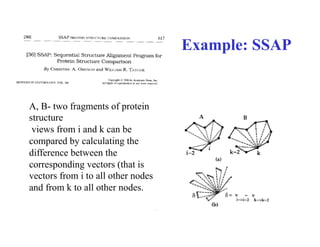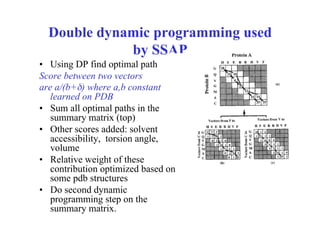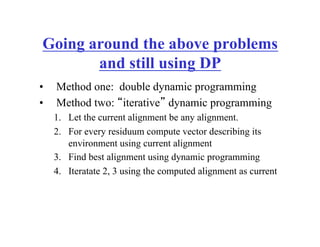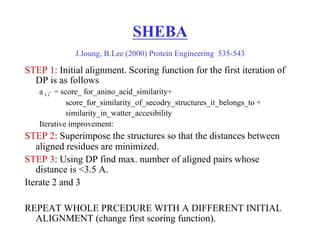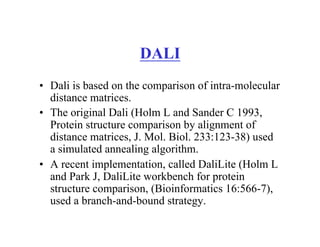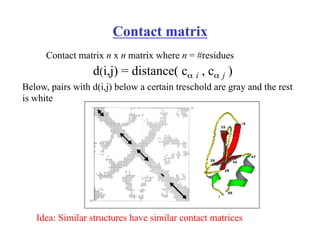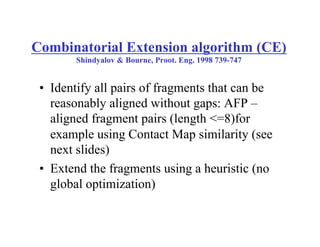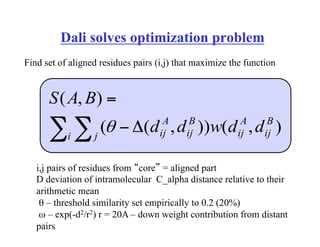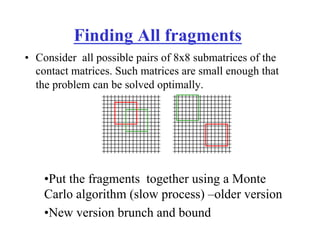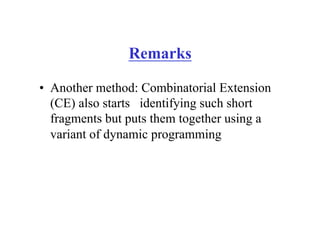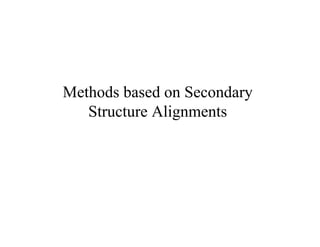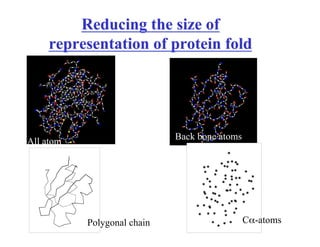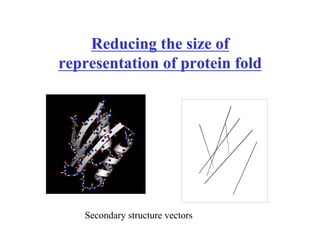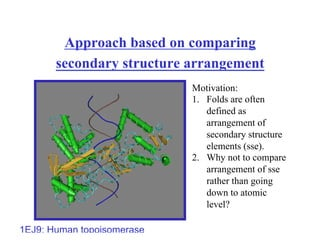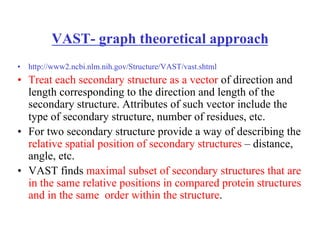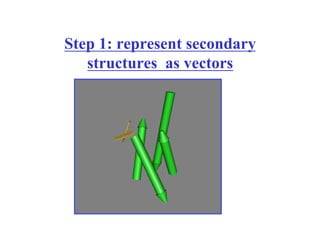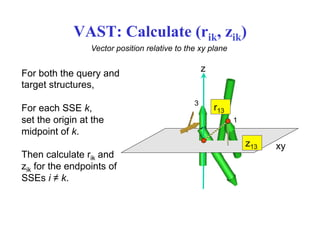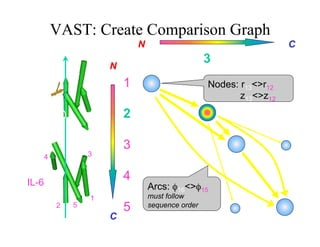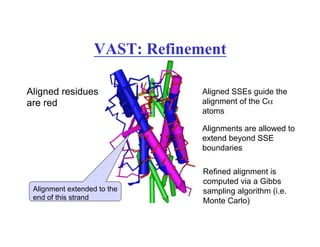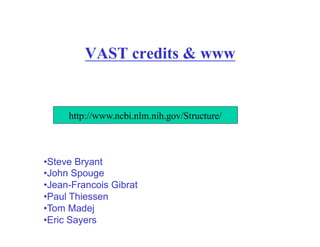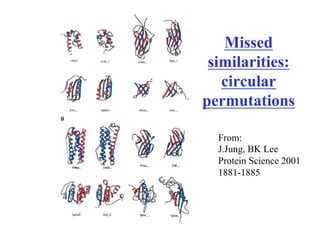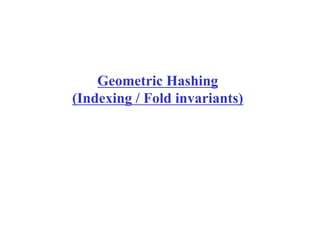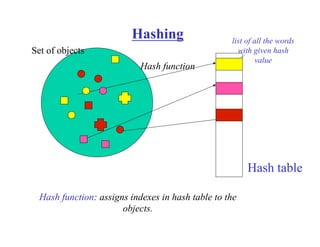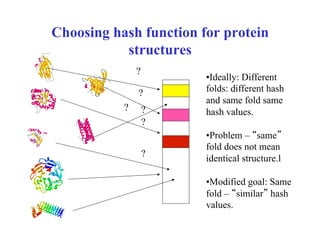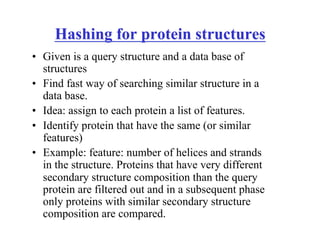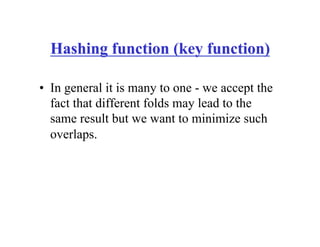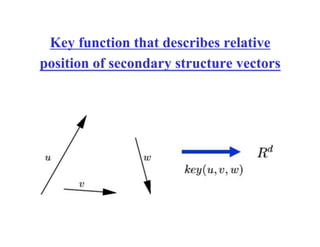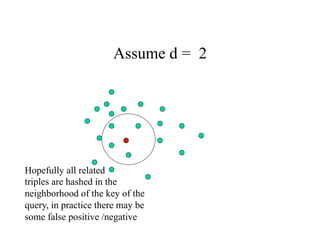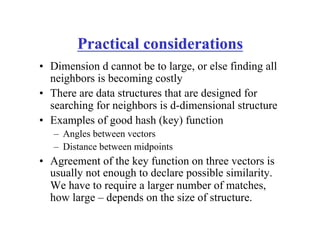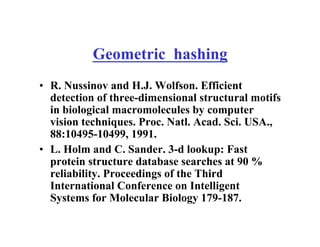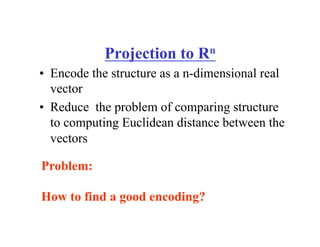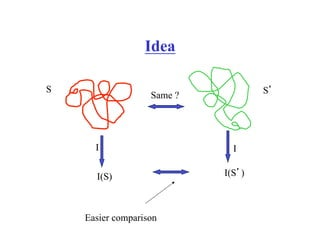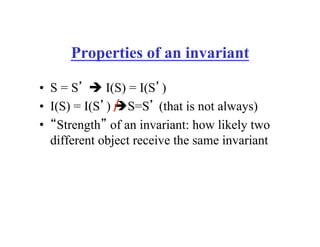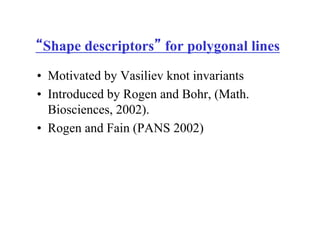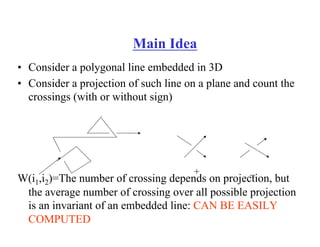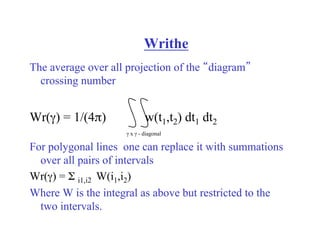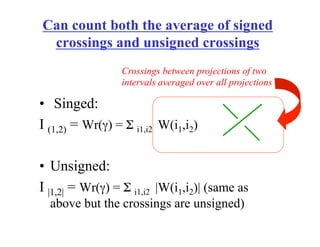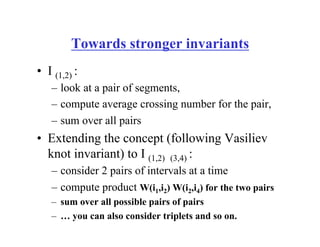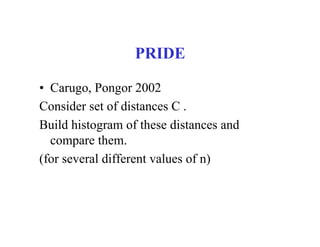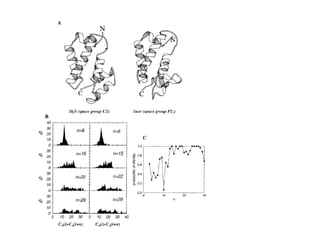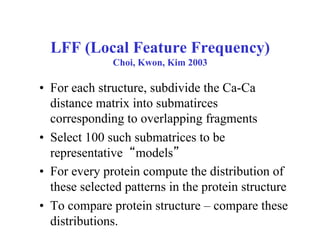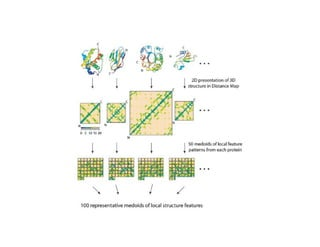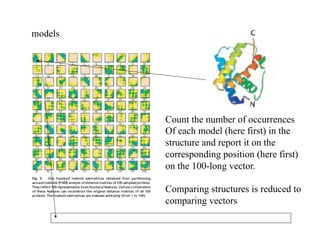1 sur 52
Publicité

### So sánh cấu trúc protein_Protein structure comparison

1. Lecture 6: Protein Structure comparison Computational Aspects of Molecular Structure Instructor: Teresa Przytycka, PhD
2. •  In evolution, structure is better preserved than sequence •  Structure comparison gives a powerful method for searching for homologous proteins . •  Structure comparison allow to study protein evolution •  To classify structures Why compare structures?
3. Superposition of two structures
4. Structural similarity between Acetylcholinesterase and Calmodulin (Tsigelny et al, Prot Sci, 2000, 9:180
5. Estimating Quality of the alignment: Root Mean Square Distance (RMSD) ∑= = N i ii bad N BARMS 1 2 ' ),( 1 ),( A= a1 … an ; B= b1 … bm ; Assume that ai is aligned with bi’ ; d(ai,bi) is the Euclidian distance between ai and bi.
6. Problems with RMSD A small local alignment error can propagate and the quality of alignment nay be underestimated
7. Finding maximum common substructure is NP hard Goal: Find the maximum subset of dots that are in both sets in the same relative position We can superimpose 6 points NP – hard: only exponential time algorithms are known
8. Methods •  Dynamic programming similar to sequence alignment (we will discuss potential problems) •  Identify pairs of fragments (usually secondary structures) that are similar and try to glue them together into consistent alignment •  Presenting it as an optimization problem and using algorithms as simulated annealing, brunch and bound etc. •  Fast screening methods to that filter structure pairs to be compared by more elaborate algorithms
9. Dynamic programming and it’s limitations •  This is not a clean dynamic programming type of problem but some program (e.g. SSAP) use DP as heuristic approach. •  Idea: score for a pair of two aligned residues is computed based on whether they are in the same context with respect to their (3D) environment. •  Environment is defined by the proximity to other close residues A, B have similar environments thus we aligned them …but… After we remove x similarity is lost We don’t know what are your neighbors until you do whole alignment! A B x y z
10. Example: SSAP A, B- two fragments of protein structure views from i and k can be compared by calculating the difference between the corresponding vectors (that is vectors from i to all other nodes and from k to all other nodes.
11. Double dynamic programming used by SSAP •  Using DP find optimal path Score between two vectors are a/(b+δ) where a,b constant learned on PDB •  Sum all optimal paths in the summary matrix (top) •  Other scores added: solvent accessibility, torsion angle, volume •  Relative weight of these contribution optimized based on some pdb structures •  Do second dynamic programming step on the summary matrix.
12. Going around the above problems and still using DP •  Method one: double dynamic programming •  Method two: “iterative” dynamic programming 1.  Let the current alignment be any alignment. 2.  For every residuum compute vector describing its environment using current alignment 3.  Find best alignment using dynamic programming 4.  Iteratate 2, 3 using the computed alignment as current
13. SHEBA J.Joung, B.Lee (2000) Protein Engineering 535-543 STEP 1: Initial alignment. Scoring function for the first iteration of DP is as follows a i i’ = score_ for_anino_acid_similarity+ score_for_similarity_of_secodry_structures_it_belongs_to + similarity_in_watter_accesibility Iterative improvement: STEP 2: Superimpose the structures so that the distances between aligned residues are minimized. STEP 3: Using DP find max. number of aligned pairs whose distance is <3.5 A. Iterate 2 and 3 REPEAT WHOLE PRCEDURE WITH A DIFFERENT INITIAL ALIGNMENT (change first scoring function).
14. DALI •  Dali is based on the comparison of intra-molecular distance matrices. •  The original Dali (Holm L and Sander C 1993, Protein structure comparison by alignment of distance matrices, J. Mol. Biol. 233:123-38) used a simulated annealing algorithm. •  A recent implementation, called DaliLite (Holm L and Park J, DaliLite workbench for protein structure comparison, (Bioinformatics 16:566-7), used a branch-and-bound strategy.
15. Contact matrix d(i,j) = distance( cα i , cα j ) Idea: Similar structures have similar contact matrices Contact matrix n x n matrix where n = #residues Below, pairs with d(i,j) below a certain treschold are gray and the rest is white
16. Combinatorial Extension algorithm (CE) Shindyalov & Bourne, Proot. Eng. 1998 739-747 •  Identify all pairs of fragments that can be reasonably aligned without gaps: AFP – aligned fragment pairs (length <=8)for example using Contact Map similarity (see next slides) •  Extend the fragments using a heuristic (no global optimization)
17. Dali solves optimization problem ),()),(( ),( B ij A ij B iji j A ij ddwdd BAS ∑ ∑ Δ− = θ i,j pairs of residues from “core” = aligned part D deviation of intramolecular C_alpha distance relative to their arithmetic mean θ – threshold similarity set empirically to 0.2 (20%) ω – exp(-d2/r2) r = 20A – down weight contribution from distant pairs Find set of aligned residues pairs (i,j) that maximize the function
18. Finding All fragments •  Consider all possible pairs of 8x8 submatrices of the contact matrices. Such matrices are small enough that the problem can be solved optimally. • Put the fragments together using a Monte Carlo algorithm (slow process) –older version • New version brunch and bound
19. Remarks •  Another method: Combinatorial Extension (CE) also starts identifying such short fragments but puts them together using a variant of dynamic programming
20. Methods based on Secondary Structure Alignments
21. Reducing the size of representation of protein fold All atom Back bone atoms Polygonal chain Cα-atoms
22. Reducing the size of representation of protein fold Secondary structure vectors
23. Approach based on comparing secondary structure arrangement Motivation: 1.  Folds are often defined as arrangement of secondary structure elements (sse). 2.  Why not to compare arrangement of sse rather than going down to atomic level? 1EJ9: Human topoisomerase I
24. VAST- graph theoretical approach •  http://www2.ncbi.nlm.nih.gov/Structure/VAST/vast.shtml •  Treat each secondary structure as a vector of direction and length corresponding to the direction and length of the secondary structure. Attributes of such vector include the type of secondary structure, number of residues, etc. •  For two secondary structure provide a way of describing the relative spatial position of secondary structures – distance, angle, etc. •  VAST finds maximal subset of secondary structures that are in the same relative positions in compared protein structures and in the same order within the structure.
25. Step 1: represent secondary structures as vectors
26. VAST: Calculate (rik, zik) 3 1 z For both the query and target structures, For each SSE k, set the origin at the midpoint of k. Then calculate rik and zik for the endpoints of SSEs i ≠ k. Vector position relative to the xy plane xyz13 r13
27. VAST: Create Comparison Graph IL-4 IL-6 3 1 4 6 1 2 3 5 1 2 3 4 5 6 1 2 3 4 5 4 2 5 Nodes: r13<>r12 z13<>z12 Arcs: φ16<>φ15 must follow sequence order Select path with highest “weights” N N C C
28. VAST: Refinement Aligned residues are red Alignment extended to the end of this strand Aligned SSEs guide the alignment of the Cα atoms Alignments are allowed to extend beyond SSE boundaries Refined alignment is computed via a Gibbs sampling algorithm (i.e. Monte Carlo)
29. VAST credits & www http://www.ncbi.nlm.nih.gov/Structure/ • Steve Bryant • John Spouge • Jean-Francois Gibrat • Paul Thiessen • Tom Madej • Eric Sayers
30. Missed similarities: circular permutations From: J.Jung, BK Lee Protein Science 2001 1881-1885
31. Geometric Hashing (Indexing / Fold invariants)
32. Hashing Hash table Hash function: assigns indexes in hash table to the objects. Hash function list of all the words with given hash value Set of objects
33. Choosing hash function for protein structures ? ? ? ? ? ? • Ideally: Different folds: different hash and same fold same hash values. • Problem – “same” fold does not mean identical structure.l • Modified goal: Same fold – “similar” hash values.
34. Hashing for protein structures •  Given is a query structure and a data base of structures •  Find fast way of searching similar structure in a data base. •  Idea: assign to each protein a list of features. •  Identify protein that have the same (or similar features) •  Example: feature: number of helices and strands in the structure. Proteins that have very different secondary structure composition than the query protein are filtered out and in a subsequent phase only proteins with similar secondary structure composition are compared.
35. Hashing function (key function) •  In general it is many to one - we accept the fact that different folds may lead to the same result but we want to minimize such overlaps.
36. Key function that describes relative position of secondary structure vectors
37. Assume d = 2 Hopefully all related triples are hashed in the neighborhood of the key of the query, in practice there may be some false positive /negative
38. Practical considerations •  Dimension d cannot be to large, or else finding all neighbors is becoming costly •  There are data structures that are designed for searching for neighbors is d-dimensional structure •  Examples of good hash (key) function –  Angles between vectors –  Distance between midpoints •  Agreement of the key function on three vectors is usually not enough to declare possible similarity. We have to require a larger number of matches, how large – depends on the size of structure.
39. Geometric hashing •  R. Nussinov and H.J. Wolfson. Efficient detection of three-dimensional structural motifs in biological macromolecules by computer vision techniques. Proc. Natl. Acad. Sci. USA., 88:10495-10499, 1991. •  L. Holm and C. Sander. 3-d lookup: Fast protein structure database searches at 90 % reliability. Proceedings of the Third International Conference on Intelligent Systems for Molecular Biology 179-187.
40. Projection to Rn •  Encode the structure as a n-dimensional real vector •  Reduce the problem of comparing structure to computing Euclidean distance between the vectors Problem: How to find a good encoding?
41. Idea Same ? S S’ I I I(S) I(S’) Easier comparison
42. Properties of an invariant •  S = S’ ! I(S) = I(S’) •  I(S) = I(S’) !S=S’ (that is not always) •  “Strength” of an invariant: how likely two different object receive the same invariant
43. “Shape descriptors” for polygonal lines •  Motivated by Vasiliev knot invariants •  Introduced by Rogen and Bohr, (Math. Biosciences, 2002). •  Rogen and Fain (PANS 2002)
44. Main Idea •  Consider a polygonal line embedded in 3D •  Consider a projection of such line on a plane and count the crossings (with or without sign) W(i1,i2)=The number of crossing depends on projection, but the average number of crossing over all possible projection is an invariant of an embedded line: CAN BE EASILY COMPUTED + _
45. The average over all projection of the “diagram” crossing number Wr(γ) = 1/(4π) w(t1,t2) dt1 dt2 For polygonal lines one can replace it with summations over all pairs of intervals Wr(γ) = Σ i1,i2 W(i1,i2) Where W is the integral as above but restricted to the two intervals. Writhe γ x γ - diagonal
46. Can count both the average of signed crossings and unsigned crossings •  Singed: I (1,2) = Wr(γ) = Σ i1,i2 W(i1,i2) •  Unsigned: I |1,2| = Wr(γ) = Σ i1,i2 |W(i1,i2)| (same as above but the crossings are unsigned) Crossings between projections of two intervals averaged over all projections
47. Towards stronger invariants •  I (1,2) : –  look at a pair of segments, –  compute average crossing number for the pair, –  sum over all pairs •  Extending the concept (following Vasiliev knot invariant) to I (1,2) (3,4) : –  consider 2 pairs of intervals at a time –  compute product W(i1,i2) W(i2,i4) for the two pairs –  sum over all possible pairs of pairs –  … you can also consider triplets and so on.
48. PRIDE •  Carugo, Pongor 2002 Consider set of distances C . Build histogram of these distances and compare them. (for several different values of n)
49. LFF (Local Feature Frequency) Choi, Kwon, Kim 2003 •  For each structure, subdivide the Ca-Ca distance matrix into submatirces corresponding to overlapping fragments •  Select 100 such submatrices to be representative “models” •  For every protein compute the distribution of these selected patterns in the protein structure •  To compare protein structure – compare these distributions.
50. models Count the number of occurrences Of each model (here first) in the structure and report it on the corresponding position (here first) on the 100-long vector. Comparing structures is reduced to comparing vectors
Publicité